# RD Sharma Solutions For Class 12 Maths Exercise 4.13 Chapter 4 Inverse Trigonometric Functions

RD Sharma Solutions for Class 12 Maths Exercise 4.13 of Chapter 4 Inverse Trigonometric Functions in PDF are available here. Students can refer and download it from the available links. This exercise has four main questions. RD Sharma Solutions for Class 12 provides solutions for all topics covered in this exercise. This exercise deals with the properties of inverse trigonometric functions with stepwise illustrations.

## Download the PDF of RD Sharma Solutions For Class 12 Chapter 4 – Inverse Trigonometric Functions Exercise 4.13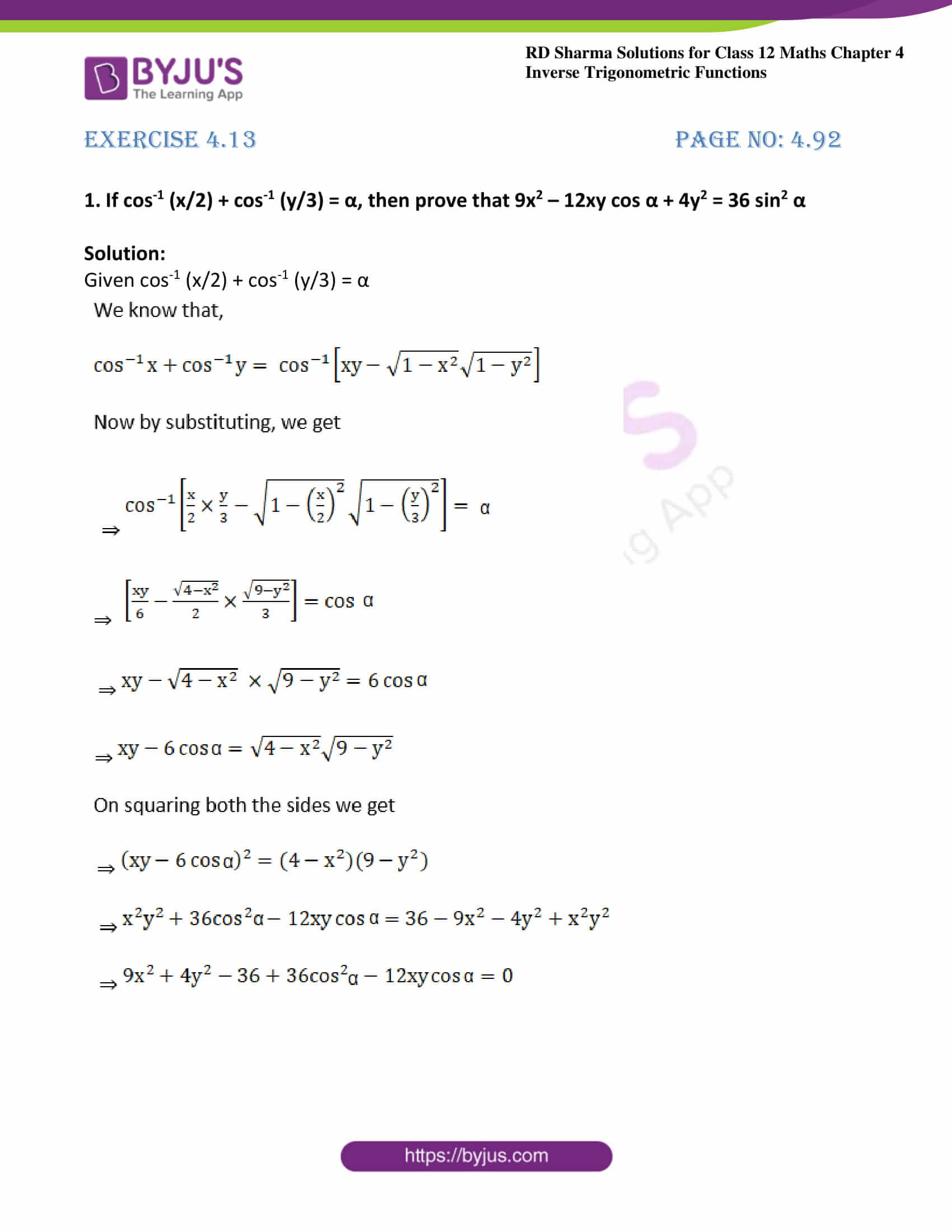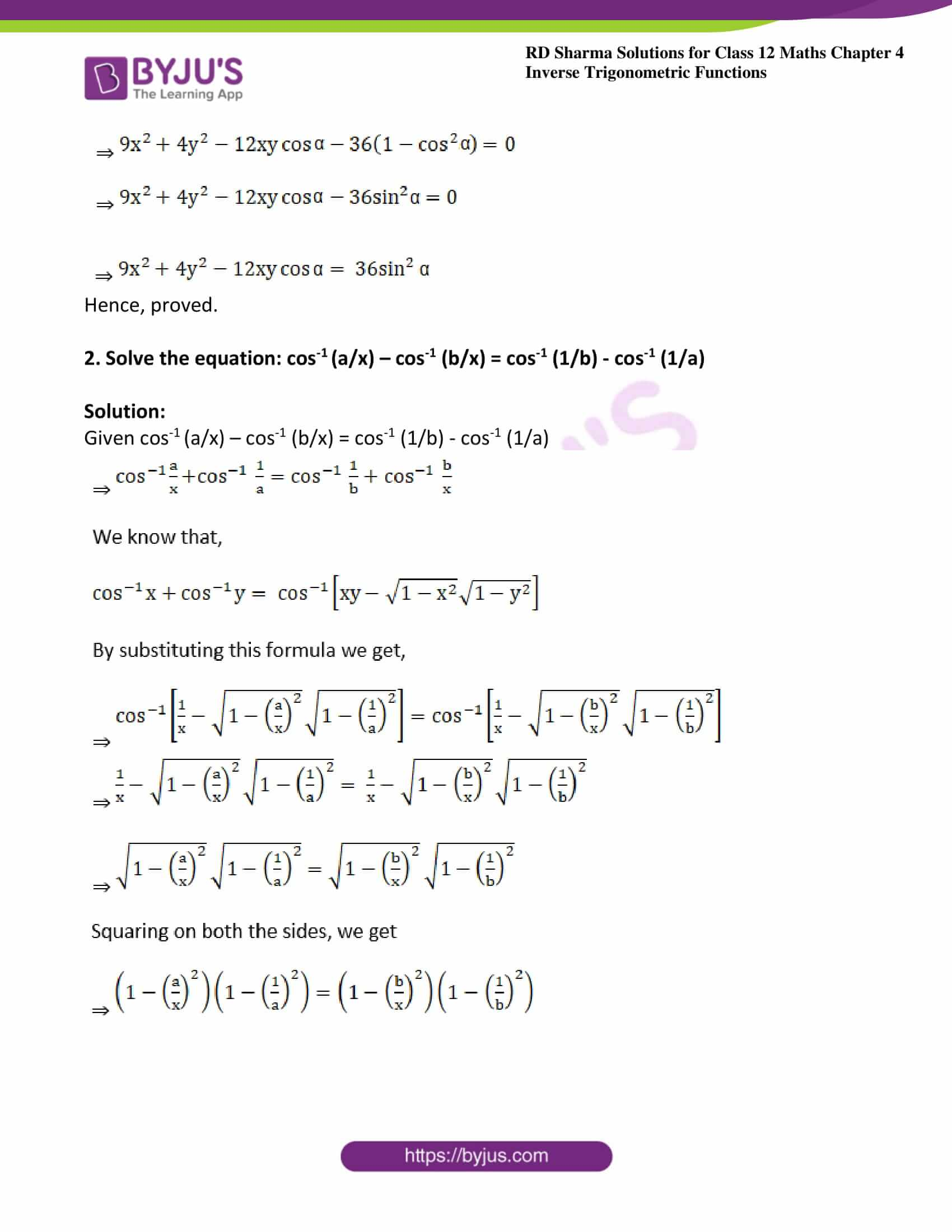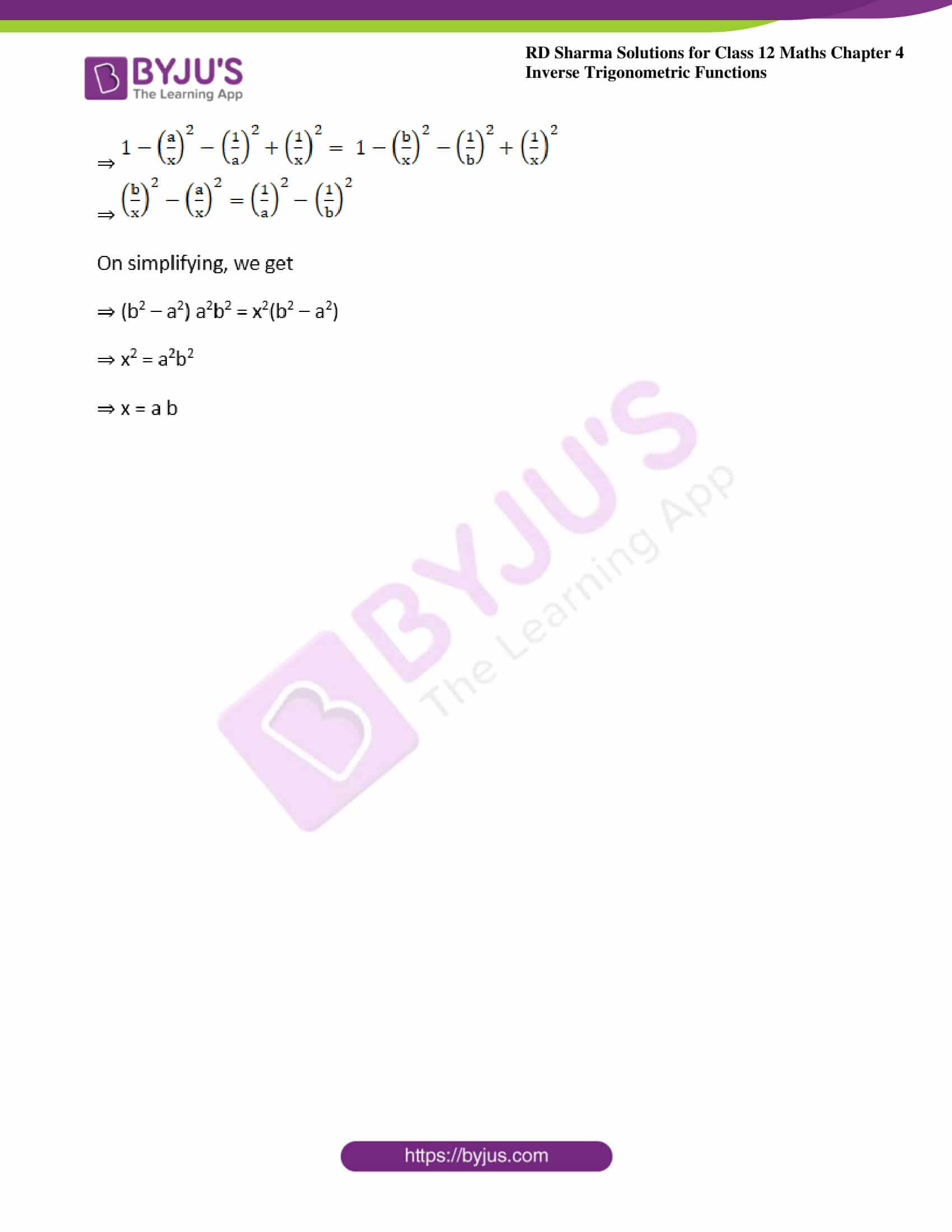### Exercise 4.13 Page No: 4.92

1. If cos-1 (x/2) + cos-1 (y/3) = α, then prove that 9x2 – 12xy cos α + 4y2 = 36 sin2 α

Solution:

Given cos-1 (x/2) + cos-1 (y/3) = α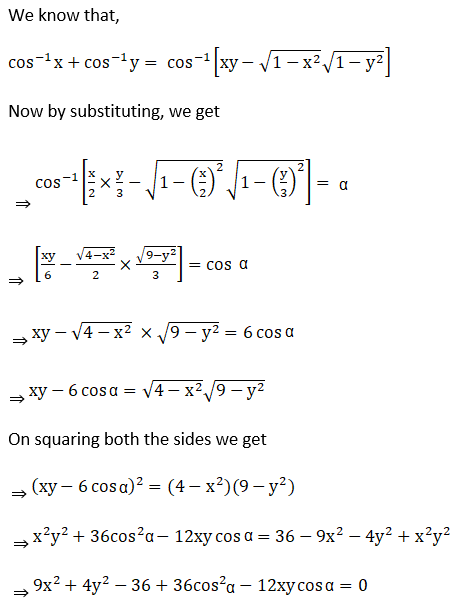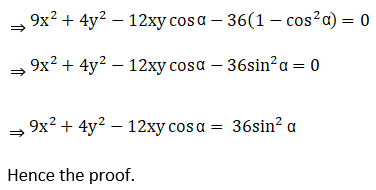Hence, proved.

2. Solve the equation: cos-1 (a/x) – cos-1 (b/x) = cos-1 (1/b) – cos-1 (1/a)

Solution:

Given cos-1 (a/x) – cos-1 (b/x) = cos-1 (1/b) – cos-1 (1/a)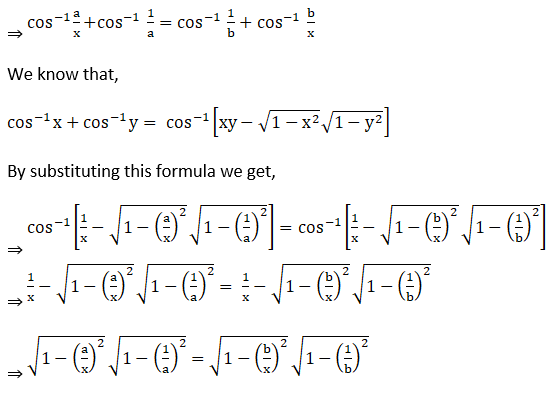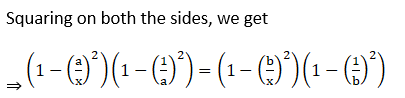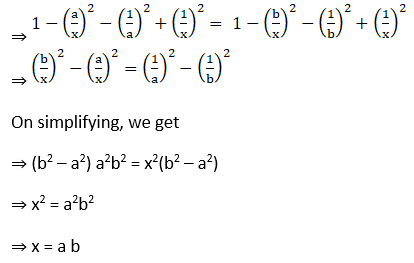### Access other exercises of RD Sharma Solutions For Class 12 Chapter 4 – Inverse Trigonometric Functions

Exercise 4.1 Solutions

Exercise 4.2 Solutions

Exercise 4.3 Solutions

Exercise 4.4 Solutions

Exercise 4.5 Solutions

Exercise 4.6 Solutions

Exercise 4.7 Solutions

Exercise 4.8 Solutions

Exercise 4.9 Solutions

Exercise 4.10 Solutions

Exercise 4.11 Solutions

Exercise 4.12 Solutions

Exercise 4.14 Solutions